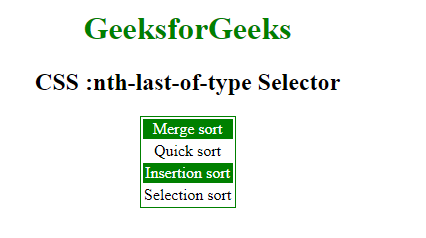# CSS | :nth-last-of-type() Selector

• Last Updated : 23 Aug, 2022

The :nth-last-of-type() Selector in CSS is used to style only those elements which are the nth number of child of the parent element. The selector counting from the last-child element. A n could be a number, a keyword, or a formula.

Syntax:

```:nth-last-of-type(number) {
//css Property;
} ```

Where number is the argument that represents the pattern for matching elements counting from the end. It can be odd, even or in a functional notation.

• odd: It represents elements whose position is odd in a series: 1, 3, 5, etc., counting from the end.
• even: It represents the elements whose position is even in a series: 2, 4, 6, etc., counting from the end.
• functional notation (): It represents elements whose position of siblings matches the pattern An+B, for every positive integer or zero value of n. The index of the first element, counting from the end, is 1.

Example-1:

## html

 ```<``html``>` `<``head``>``    ``<``style``>``        ``h1 {``            ``color: green;``            ``font-size: 35px;``        ``}``        ` `        ``p:nth-last-of-type(3) {``            ``background: green;``            ``color: white;``            ``font-weight: bold;``            ``width: 70%;``        ``}``    ````` `<``body``>``    ``<``center``>``        ``<``h1``>GeeksForGeeks``        ``<``h2``>CSS:nth-last-of-type() Selector ``        ``<``p` `class="geeksFORGEEKS">geeksforgeeks``            ``<``p` `class="forgeeks">A computer science portal for geeks.``                ``<``p` `class="geeks">Sudo placement.``                ``<``p` `class="SUDO">GFG STANDS FOR GEEKSFORGEEKS.` `` `               `

Output :Example-2: In this example every even element is selected according to formula 2n counting from the end.

## html

 ```<``html``>` `<``head``>``    ``<``title``>CSS :nth-last-of-type Selector``    ``<``style``>``        ``table {``            ``border: 1px solid green;``            ``margin: auto;``        ``}``        ``/* Selects the last three element */``        ` `        ``tr:nth-last-of-type(2n) {``            ``background-color: green;``            ``color: white;``        ``}``    ````` `<``body` `style="text-align:center;">` `    ``<``h1` `style="color:green;">``            ``GeeksforGeeks``        ``` `    ``<``h2``>``            ``CSS :nth-last-of-type Selector``        ``` `    ``<``table``>``        ``<``tbody``>``            ``<``tr``>``                ``<``td``>Merge sort``            ````            ``<``tr``>``                ``<``td``>Quick sort``            ````            ``<``tr``>``                ``<``td``>Insertion sort``            ````            ``<``tr``>``                ``<``td``>Selection sort``            ````        ````    ``` `` ``

Output :Supported Browsers: The browser supported by :nth-last-of-type() Selector are listed below:

• Apple Safari 3.1
• Google Chrome 4
• Edge 12
• Firefox 3.5
• Opera 9.5

My Personal Notes arrow_drop_up